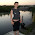Detection of Outliers in Analytical Data – The Grubb’s Test | Chemistry Net

# Detection of Outliers in Analytical Data – The Grubb’s Test

Many statistical techniques used for the treatment of quantitative data are sensitive to the presence of outliers. Simple calculations such as the calculation of the mean and standard deviation of a set of data may be distorted by even an outlying point. Checking therefore for outliers should be a routine part of any data analysis.
A commonly used statistical test is the Dixon’s Q-test we presented in a previous post entitled “Detection of a Single Outlier|StatisticalAnalysis|Quantitative Data”.
Another similar but more robust test for the detection of outliers is the Grubb’s test. It is now considered as a more accurate test than Dixon’s Q-test.

The Grubb’s test1 is used to detect a single outlier in a data set of N values that are nearly normally distributed. This test is essentially based on the criterion of “distance of the suspected value from the mean of the data set compared with the standard deviation”.
The test is performed by computing the Grubbs G which is defined as:

Gexp = |xoutlier - x̅| / s                         (1)

Where:
xoutlier is the suspected outlier
x̅ is the mean of the N values
s is the standard deviation of N values

If the calculated Gexp is found to be:
• Gexp  <  G  then the point in question must be retained
•  Gexp  >  G  then the point in question must be discarded and the mean and standard deviation must be recalculated.

Where G is found from statistical tables (see Table I.1) for different levels of confidence and number of data points.

How the Grubb’s test is applied?

The test is very simple and it is applied as follows:

•      Order the N data values comprising the set of observations under examination in increasing order:
x1 < x2 < x3< xN

•       Calculate the average of the data values x̅ and the standard deviation s
•        Calculate the experimental Gexp. Gexp is defined  in equation (1)
•        The value of Gexp is compared with a critical value of Gcritical found in tables. The critical value should correspond to the confidence level we have decided to run the test (usually 95% confidence).
If the calculated Gexp is found to be:

1)   Gexp  <  Gcritical  then the point in question must be retained
2)   Gexp  >  Gcritical  then the point in question must be discarded and the mean and standard deviation must be recalculated.

A table containing Gcritical values for different confidence levels (95%, 97.5%, 99%) and
number of data N (3-100) is given below:

Table I.1: Critical values of G-test1

 N Gcritical (95%)** Gcritical (97.5%)** Gcritical (99%)** 3 1.15 1.15 1.15 4 1.46 1.48 1.49 5 1.67 1.71 1.75 6 1.82 1.89 1.94 7 1.94 2.02 2.10 8 2.03 2.13 2.22 9 2.11 2.21 2.32 10 2.18 2.29 2.41 11 2.23 2.36 2.48 12 2.29 2.41 2.55 13 2.33 2.46 2.61 14 2.37 2.51 2.66 15 2.41 2.55 2.71 16 2.44 2.59 2.75 17 2.47 2.62 2.79 18 2.50 2.65 2.82 19 2.53 2.68 2.85 20 2.56 2.71 2.88 21 2.58 2.73 2.91 22 2.60 2.76 2.94 23 2.62 2.78 2.96 24 2.64 2.80 2.99 25 2.66 2.82 3.01 30 2.75 2.91 35 2.82 2.98 40 2.87 3.04 45 2.92 3.09 50 2.96 3.13 60 3.03 3.20 70 3.09 3.26 80 3.14 3.31 90 3.18 3.35 100 3.21 3.38

** The percentage expresses the confidence level.

Are there any limitations to Grubb’s Test?

1.  The data excluding  the possible outlier must be nearly normally distributed (use the Normal Q-Q plot)
2.   The Grubb’s-test is valid for the detection of a single outlier (it cannot be used for a second time on the same set of data).
3.   The Grubb’s test should be applied with caution – the same applies to all statistical tests used for rejecting data - since there is a probability, equal to the significance level a (a = 0.05 at the 95% confidence level) that an outlier identified by the Grubb’s-test actually is not an outlier.
4.   The mean and the standard deviation s of the values in the data set must be calculated - in cases where it is desirable to avoid the calculation of standard deviation or where quick judgment is called for the Dixon’s Q-test may be used instead.

A typical example with a possible outlier value was given in a previous post entitled “Calibration and
Can we reject the 0.6400 value (please see Table I.1 in “Calibrationand Outliers - Statistical
Analysis”)  as an outlier at a 95% confidence level  using Grubbs-test?

By following the above procedure we get the following:
The data excluding  the possible outlier are almost normally distributed as shown in  Fig. 1b
in “Calibration and Outliers - Statistical Analysis”
Arrange the data under examination in increasing order:

0.5980  0.5993  0.5995  0.5997  0.601  0.6400

Calculate the mean of the data values and the standard deviation:

x̅ = 0.6062,   s = 0.0166

Calculate Gexp using equation (1):
Gexp = |0.6400 – 0.6062| / 0.0166  = 2.04
Compare with the critical value of Gcritical found in table I.1 at the 95% confidence
level and for N = 6 observations. This value is equal to Gcritical = 1.82
Gexp = 2.04 > Gcritical = 1.82 and therefore we can reject 0.6400 at the 95% confidence
level being certain that there is a probability a < 0.05 that our decision is false.
In a previous post the Dixon’s Q-test also showed that the value 0.6400 is an outlier.

References

1.      F. E Grubbs,  Technometrics, 11, 1–21, (1969)

1.1.2.